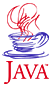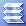Home : Course Map : Chapter 9 : Java : File Binary I/OIntroduction Overview Streams Wrappers,Buffers Console I/O   Text Output       Demo 1   Formatter/printf()      Demo 2   Tex 2t Input      Demo 3   Scanner      Demo 4 File Class   File I/O   File Output-Text      Demo 5   Formatter to File      Demo 6   File Input - Text     Demo 7   Scanner - Files      Demo 8   File I/O - Binary      Demo 9   Demo 10 File Chooser Dialog   Demo 11 Character Codes   Demo 12   Demo13 Object I/O Types to Bytes Stream Filters Other I/O Topics Exercises
 Supplements Character I/O   Demo 1   Demo 2 Random Access   Demo 3 ZIP/GZIP Streams   Demo 4 Piped Streams   Demo 5 NIO Framework More NIO   Demo 6
 About JavaTech      Codes List      Exercises      Feedback      References      Resources      Tips      Topic Index      Course Guide      What's New

Numerical data transfers faster and more compactly in a raw binary format than as text characters. Here we look at examples of writing numerical data to a binary file and reading numerical data from a binary file.

Data transfers faster and more compactly in binary than as text characters. Here we look at examples of writing numerical data to a binary file and reading numerical data from a file.

Writing to a Binary File

In the example program below called BinOutputFileApp, we first create some data arrays with some arbitrary values. We then open a stream to a file with the binary FileOutputStream class. We wrap this stream object with an instance of the DataOutputStream class, which contains many useful methods for writing primitive types of the writeX() form, where X indicates a primitive type.

We use the writeInt (int i) and the writeDouble (double d) methods, to write the data to the file as pairs of int/double type values. In the next subsection below, we will show next how to read the binary data from this file.

 BinOutputFileApp.java Resources: numerical.dat import java.io.*; import java.util.*; /**  Write a primitive type data array to a binary file.**/ public class BinOutputFileApp {   public static void main (String arg[]) {     Random ran = new Random ();     // Create an integer array and a double array.     int    [] i_data = new int;     double [] d_data = new double;     // and fill them     for  (int i=0; i < i_data.length; i++) {       i_data[i] = i;       d_data[i] = ran.nextDouble () * 10.0;     }     File file = null;     // Get the output file name from the argument line.     if (arg.length > 0) file = new File (arg);     // or use a default file name     if (file == null) {         System.out.println ("Default: numerical.dat");         file = new File ("numerical.dat");     }     // Now write the data array to the file.     try {       // Create an output stream to the file.       FileOutputStream file_output = new FileOutputStream (file);       // Wrap the FileOutputStream with a DataOutputStream       DataOutputStream data_out = new DataOutputStream (file_output);       // Write the data to the file in an integer/double pair       for (int i=0; i < i_data.length; i++) {           data_out.writeInt (i_data[i]);           data_out.writeDouble (d_data[i]);       }       // Close file when finished with it..       file_output.close ();     }     catch (IOException e) {        System.out.println ("IO exception = " + e );     }   } // main } // class BinOutputFileApp

In the example program BinInputFileApp, we read a binary file created by BinOutputFileApp discussed above. We begin by first opening a stream to the file with a FileInputStream object. Then we wrap this with a DataInputStream class to obtain the many readX() methods, where X represents the name of a primitive data type as in readInt() and readDouble(). The BinInputFileApp program reads pairs of integer and double values.

Rather than test for the return of a -1 value as we did in the text input streams, we simply continue to loop until the read method throws the EOFException. In the catch statement for this exception you can carry out the final housekeeping chores before closing the file stream.

 import java.io.*; import java.util.*; /**  Demonstrate reading primitive type values from a binary file. **/ public class BinInputFileApp {   public static void main (String arg[]) {     File file = null;     int    i_data = 0;     double d_data = 0.0;     // Get the file from the argument line.     if (arg.length > 0) file = new File (arg);     if (file == null) {       System.out.println ("Default: numerical.dat");       file = new File ("numerical.dat");     }     try {       // Wrap the FileInputStream with a DataInputStream       FileInputStream file_input = new FileInputStream (file);       DataInputStream data_in    = new DataInputStream (file_input );       while (true) {         try {           i_data = data_in.readInt ();           d_data = data_in.readDouble ();         }         catch (EOFException eof) {           System.out.println ("End of File");           break;         }         // Print out the integer, double data pairs.         System.out.printf ("%3d. Data = %8.3e %n", i_data, d_data );       }       data_in.close ();     } catch  (IOException e) {        System.out.println ( "IO Exception =: " + e );     }   } // main } // class BinInputApp

We illustrate the output and input of binary data by first running BinOutputFileApp to produce the data file numerical.dat. We then run BinInputFileApp, which reads the file numerical.dat and produces the following output on the console.

Your output will vary since BinOutputFileApp uses the Random class to generate random values.

 Example Default: numerical.dat   0. Data = 2.633e+00   1. Data = 7.455e+00   2. Data = 2.447e+00   3. Data = 7.046e+00   4. Data = 2.652e+00   5. Data = 5.120e+00   6. Data = 1.754e+00   7. Data = 7.489e+00   8. Data = 7.386e-01   9. Data = 6.036e+00  10. Data = 7.002e-01  11. Data = 9.625e+00  12. Data = 5.966e+00  13. Data = 8.535e+00  14. Data = 2.744e+00 End of File

References & Web Resources

• Class specs:

Latest update: March 8, 2006
 Tech Histogram I/O Hist I/O - Get/Set   Demo 1 Hist I/O - Objects   Demo 2 HistogramStream   Demo 3 Filtering Data   Demo 4 Exercises
 Physics Physics Model Simulation Design Physics Simulator   Demo 1 Experiment Design Experiment Sim.   Demo 2 Analysis Expt. + Analysis   Demo 3 ExercisesPart I Part II Part III Java Core 1  2  3  4  5  6  7  8  9  10  11  12 13 14 15 16 17 18 19 20 21 22 23 24 Supplements 1  2  3  4  5  6  7  8  9  10  11  12 Tech 1  2  3  4  5  6  7  8  9  10  11  12 Physics 1  2  3  4  5  6  7  8  9  10  11  12

Java is a trademark of Sun Microsystems, Inc.